# RD Sharma Solutions For Class 12 Maths Exercise 6.5 Chapter 6 Determinants

RD Sharma Solutions for Class 12 Maths Exercise 6.5 Chapter 6 Determinants is provided here. The RD Sharma textbook contains examples before each exercise to improve problem-solving speed among students. These concepts are explained in simple language, which makes it possible even for a student not proficient in Maths to understand the subject better.

RD Sharma Solutions are prepared by a set of experts at BYJU’S with the aim of helping students boost their exam preparation. The solution of a homogeneous system of linear equations is the main concept covered under this exercise. In order to excel in the board exams, students can access RD Sharma Solutions for Class 12 Maths Chapter 6 Determinants Exercise 6.5.

## RD Sharma Solutions For Class 12 Chapter 6 – Determinants Exercise 6.5:-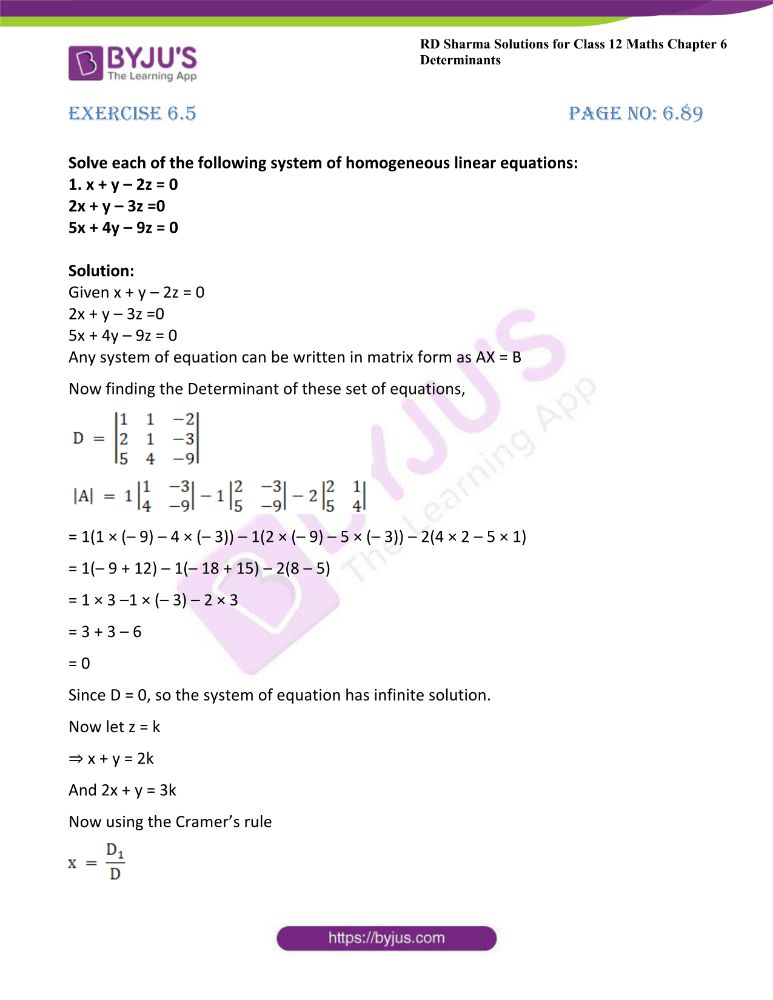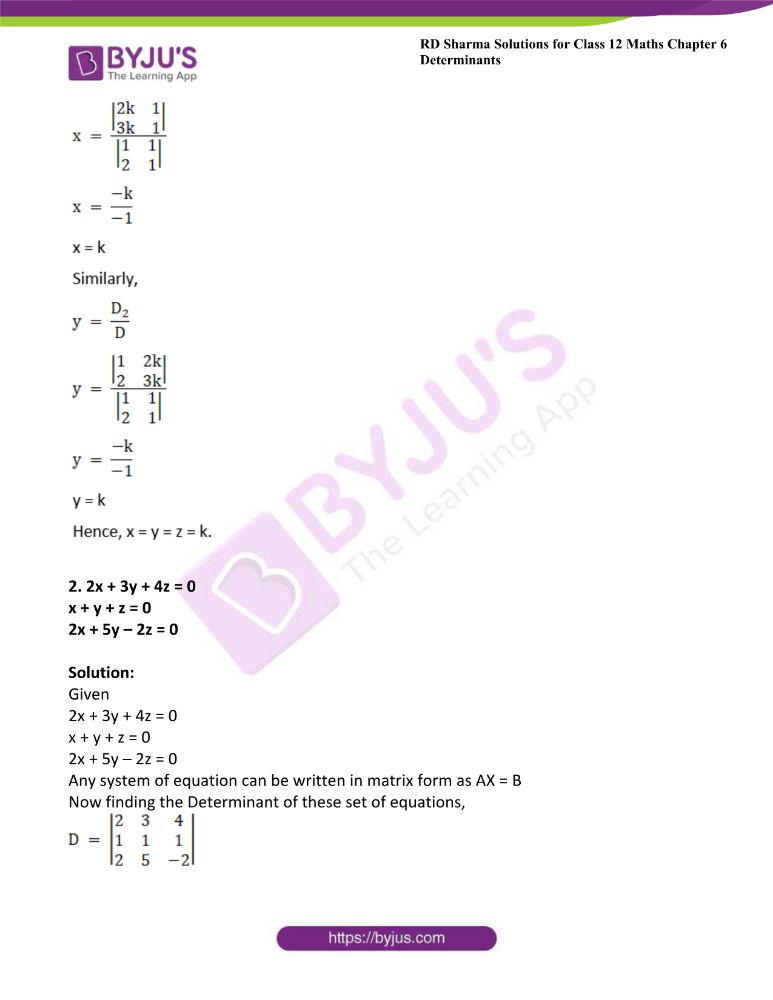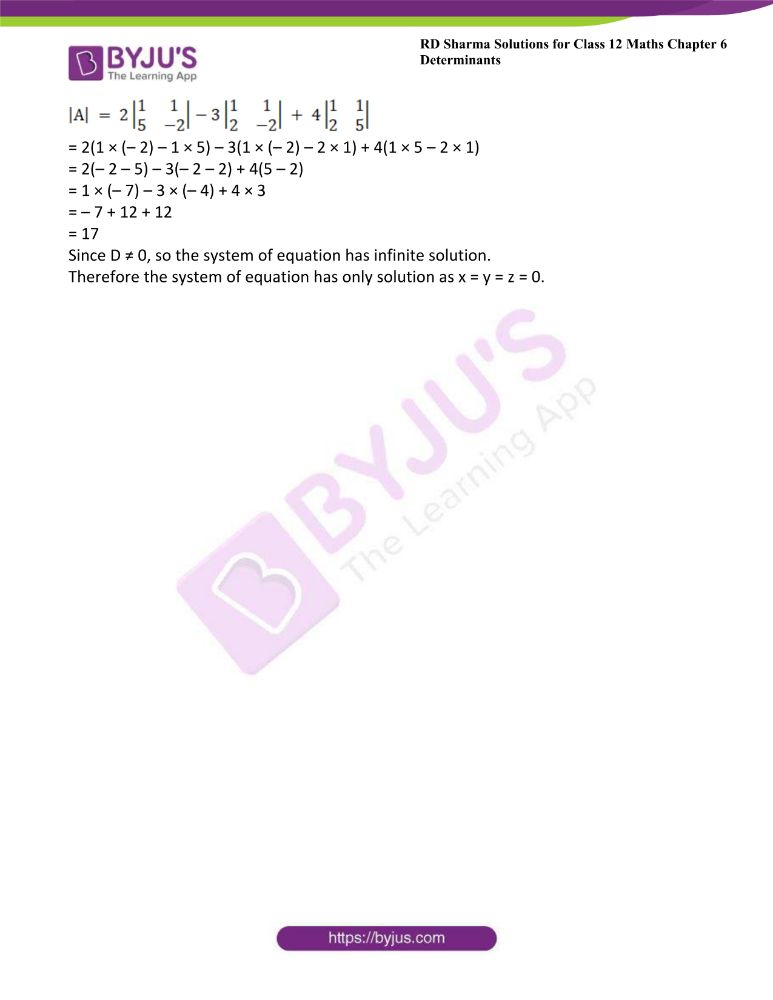### Access other exercises of RD Sharma Solutions For Class 12 Chapter 6 – Determinants

Exercise 6.1 Solutions

Exercise 6.2 Solutions

Exercise 6.3 Solutions

Exercise 6.4 Solutions

### Access answers to Maths RD Sharma Solutions For Class 12 Chapter 6 – Determinants Exercise 6.5

Exercise 6.5 Page No: 6.89

Solve each of the following system of homogeneous linear equations:

1. x + y – 2z = 0

2x + y – 3z =0

5x + 4y – 9z = 0

Solution:

Given x + y – 2z = 0

2x + y – 3z =0

5x + 4y – 9z = 0

Any system of equation can be written in matrix form as AX = B

Now finding the Determinant of these set of equations,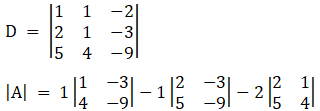= 1(1 × (– 9) – 4 × (– 3)) – 1(2 × (– 9) – 5 × (– 3)) – 2(4 × 2 – 5 × 1)

= 1(– 9 + 12) – 1(– 18 + 15) – 2(8 – 5)

= 1 × 3 –1 × (– 3) – 2 × 3

= 3 + 3 – 6

= 0

Since D = 0, so the system of equation has infinite solution.

Now let z = k

⇒ x + y = 2k

And 2x + y = 3k

Now using the Cramer’s rule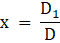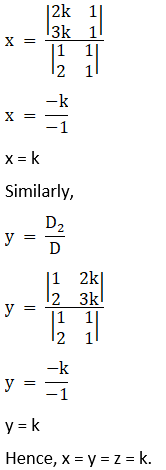2. 2x + 3y + 4z = 0

x + y + z = 0

2x + 5y – 2z = 0

Solution:

Given

2x + 3y + 4z = 0

x + y + z = 0

2x + 5y – 2z = 0

Any system of equation can be written in matrix form as AX = B

Now finding the Determinant of these set of equations,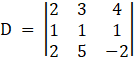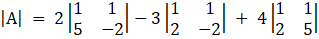= 2(1 × (– 2) – 1 × 5) – 3(1 × (– 2) – 2 × 1) + 4(1 × 5 – 2 × 1)

= 2(– 2 – 5) – 3(– 2 – 2) + 4(5 – 2)

= 1 × (– 7) – 3 × (– 4) + 4 × 3

= – 7 + 12 + 12

= 17

Since D ≠ 0, so the system of equation has infinite solution.

Therefore the system of equation has only solution as x = y = z = 0.